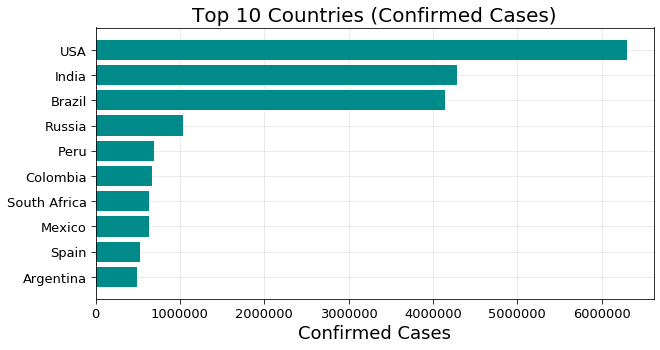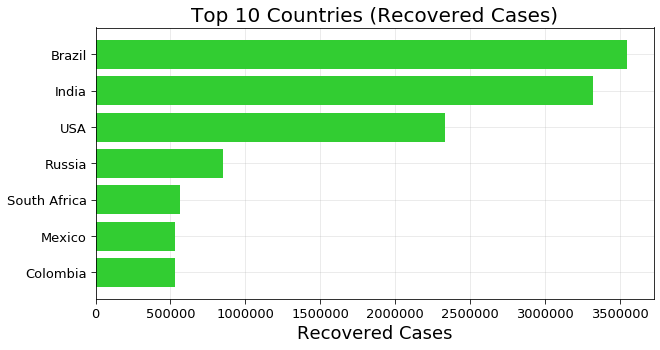Search

# Covid-19 Worldwide AnalysisIntroduction:

With the rapid spread in the novel corona-virus across countries, the World Health Organisation (WHO)and several countries have published the latest results on the impact of COVID-19 over the past few months.

I have been going through many sources and articles to understand the fatality trend and I was excited to come across this data source and decided to see some visualization on the same. The aim here is to understand how visualization helps to derive informative insights from data sources.

Dependencies

• Pandas

• Numpy

• Matplotlib

• Seaborn

• Plotly

• Folium

1. Importing The Libraries

```import pandas as pd
import numpy as np
import matplotlib.pyplot as plt
from matplotlib import ticker
import pycountry_convert as pc
import folium
import branca
from datetime import datetime, timedelta,date
from scipy.interpolate import make_interp_spline, BSpline
import plotly.express as px
import json, requests

from keras.layers import Input, Dense, Activation, LeakyReLU
from keras import models

%matplotlib inline```

In this Blog, we need to import all the libraries with all the dependencies.

The next step is to import the CSV files.

```# Retriving Dataset

The very first analysis will be the % of Active, Confirmed, and Death cases all over the world.so from this above figure, we can see there are more % of recovered than Active and Deaths, whereas deaths hold the least %.

Let's analyze Top-10 countries on the basis of Confirmed, Recovered, and Deaths.

```df_countries_cases = df_covid19.copy().drop(['Lat','Long_','continent','Last_Update'],axis =1)
df_countries_cases.index = df_countries_cases["country"]
df_countries_cases = df_countries_cases.drop(['country'],axis=1)

df_continents_cases = df_covid19.copy().drop(['Lat','Long_','country','Last_Update'],axis =1)
df_continents_cases = df_continents_cases.groupby(["continent"]).sum()

f = plt.figure(figsize=(10,5))

plt.axes(axisbelow=True)
plt.barh(df_countries_cases.sort_values('Confirmed')["Confirmed"].index[-10:],df_countries_cases.sort_values('Confirmed')["Confirmed"].values[-10:],color="darkcyan")
plt.tick_params(size=5,labelsize = 13)
plt.xlabel("Confirmed Cases",fontsize=18)
plt.title("Top 10 Countries (Confirmed Cases)",fontsize=20)
plt.grid(alpha=0.3)```From the above figure, we can conclude that the USA reports the most number of Confirmed cases In accordance with it India and Brazil report the number of confirmed cases in the second and third positions respectively.

Let's see for the Recovered and Deaths cases

```f = plt.figure(figsize=(10,5))

plt.axes(axisbelow=True)
plt.barh(df_countries_cases.sort_values('Recovered')["Recovered"].index[-10:],df_countries_cases.sort_values('Recovered')["Recovered"].values[-10:],color="limegreen")
plt.tick_params(size=5,labelsize = 13)
plt.xlabel("Recovered Cases",fontsize=18)
plt.title("Top 10 Countries (Recovered Cases)",fontsize=20)
plt.grid(alpha=0.3,which='both')```From the above figure, we can conclude that Brazil reports the most number of Recovered cases In accordance with it India and the USA reports the number of recovered cases in the second and third positions respectively.

Let's see for the Active and Deaths cases.From the above figure, we can conclude that the USA reports the most number of deaths cases In accordance with it India and Brazil report the number of Deaths cases in the second and third positions respectively.

Let's see for Active cases.From the above figure, we can conclude that the USA reports the most number of Active cases In accordance with it India and Brazil report the number of Active cases in the second and third positions respectively.The above figure itself shows the effect of Corona-virus all over the world.

Let's analyze with log-baseHere are the confirmed cases heatmap.

Let's see confirmed, Recovered, Deaths in One figure.

```confirmed = df1.groupby('ObservationDate').sum()['Confirmed'].reset_index()
deaths = df1.groupby('ObservationDate').sum()['Deaths'].reset_index()
recovered = df1.groupby('ObservationDate').sum()['Recovered'].reset_index()
fig = go.Figure()
fig.add_trace(go.Scatter(x=deaths['ObservationDate'], y=deaths['Deaths'], mode='lines+markers', name='Deaths', line=dict(color='Red', width=2)))
fig.add_trace(go.Scatter(x=recovered['ObservationDate'], y=recovered['Recovered'], mode='lines+markers', name='Recovered', line=dict(color='Green', width=2)))
fig.update_layout(title='Worldwide NCOVID-19 Cases', xaxis_tickfont_size=14,yaxis=dict(title='Number of Cases'))
fig.show()```The x-axis represents the Dates whereas the y-axis represents the number of cases

The confirmed graph shows in blue whereas the deaths and recovered are indicated as red and green color respectively.

Most affected Countries

```#COVID-19 Spread Trends in Few Most Affected Countries
df_countries = df_confirmed.groupby(["country"]).sum()
df_countries = df_countries.sort_values(df_countries.columns[-1],ascending = False)
countries = df_countries[df_countries[df_countries.columns[-1]] >= 1000].index

cols =2
rows = int(np.ceil(countries.shape/cols))
f = plt.figure(figsize=(20,8*rows))
for i,country in enumerate(countries):
visualize_covid_cases(df_confirmed, df_deaths,country = country,figure = [f,rows,cols, i+1])

plt.show()```
.png

The animation is attached below the changes and spreading of coronavirus in the respective months.

Animation
.mp4